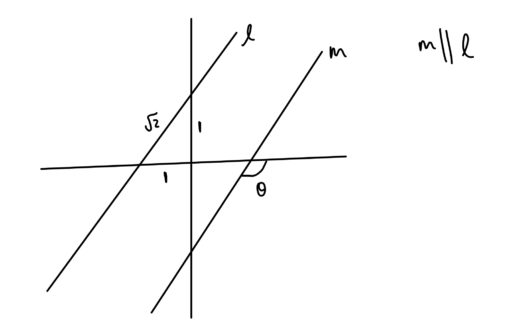Given parallel lines, there are four main relationships that angles can have to one another: vertical angles, interior angles relationships, exterior angle relationships, and corresponding angles. These relationships can all be derived from one another, and in what follows, we include some of those derivations so that, if you forget one of them on exam day, you can recover it from knowledge of the others.

Vertical Angles: Two angles formed by the same lines are vertical angles if they are on opposite sides of the point of intersection between the two lines.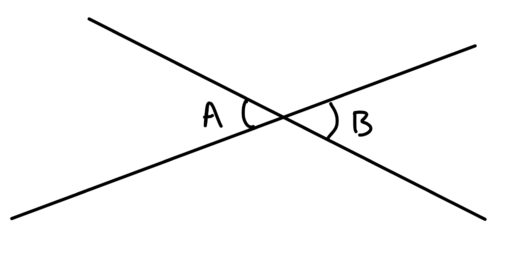This is a fundamental fact that is often critical in finding an unknown angle. For example:

Example 1: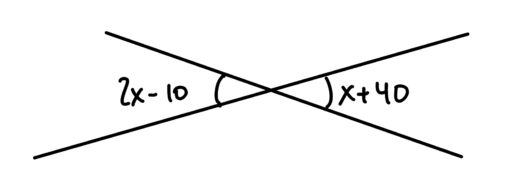Interior Angles: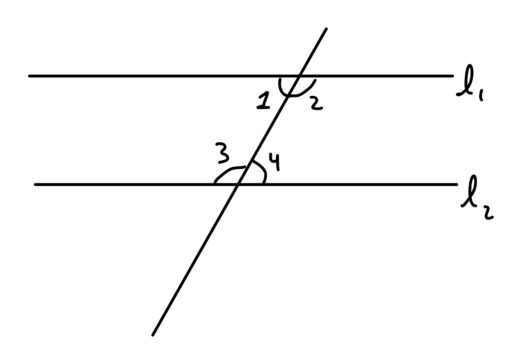In the above diagram,Example 2:

In the following diagram, letand letWhat is the value of?

Exterior Angles: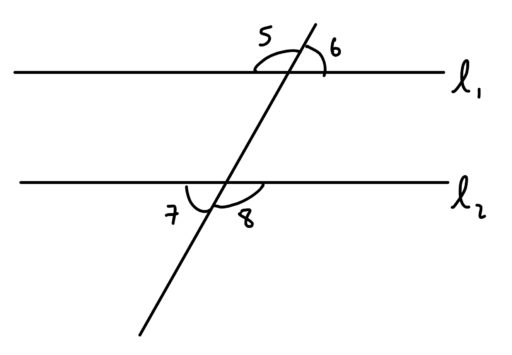In the above diagram,Example 3:

In the below diagram, letandWhat is the value of?Corresponding Angles: Two angles (which are in the same relative position) formed by a single line cutting across two parallel lines. Such angles are equal.

For example: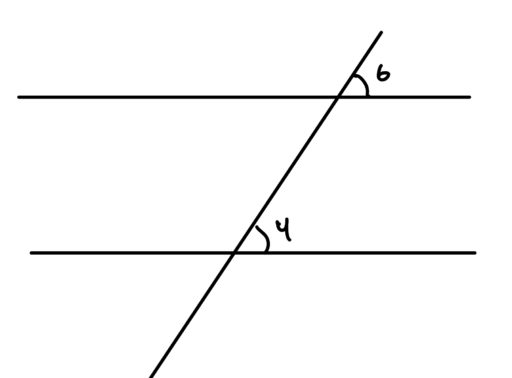In this diagram,andare corresponding angles and thus equal in degree.

Practice Problems:

1. Find the value of.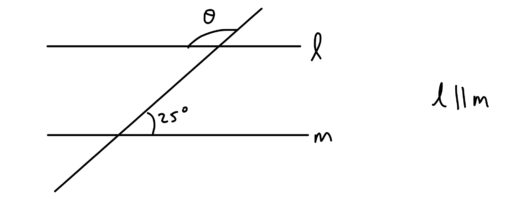2. Find the value of.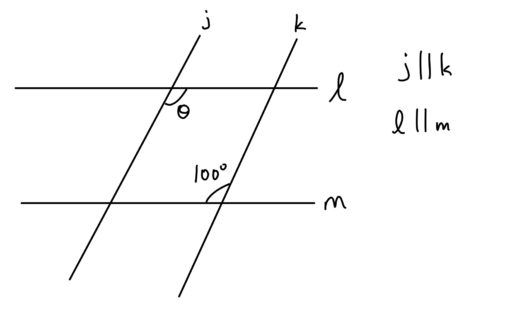3. Find the value of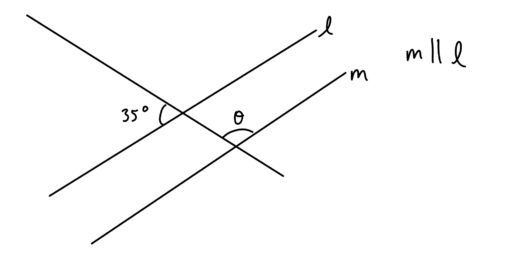4. Find the value of